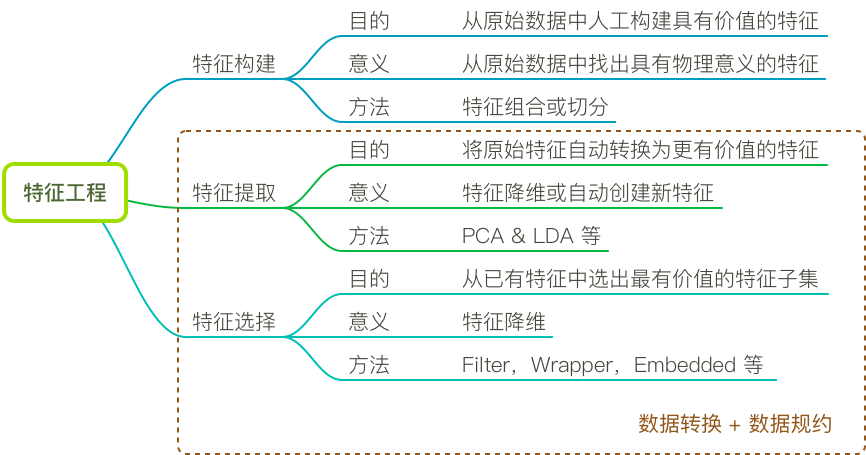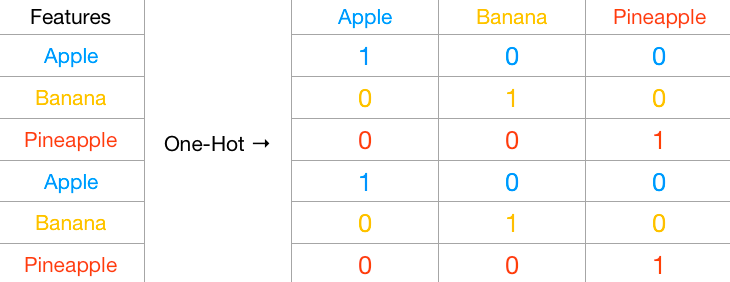## 数据分析：数据预处理之数据转换

2816 字 · 240 阅读 · 2023 年 06 月 13 日• Min-Max 标准化
• Z-Score 标准化
• 独热编码
• 数据离散化

### 特征工程概述#### 小贴士### 标准化

import numpy as np
import pandas as pd
%matplotlib inline

np.random.seed(10)  # 随机数种子
df = pd.DataFrame({'A': np.random.random(
20), 'B': np.random.random(20) * 10000})
df


df.plot()  # 绘图


### Z-Score 标准化

Z-Score 标准化 是常用的标准化手段之一，其公式为：

$$\hat x=\frac{x-\mu}{\sigma}$$

df_z_score = (df - df.mean()) / df.std()  # Z-Score 标准化
df_z_score

df_z_score.plot()


Z-Score 标准化方法在 Scipy 中有一个对应的 API scipy.stats.zscore 可供使用:

from scipy import stats

stats.zscore(df)


(df.values - df.values.mean(axis=0)) / df.values.std(axis=0)  # 将 DataFrame 处理从 NumPy 数组再运算


### Min-Max 标准化

Min-Max 标准化 同样是常用手段，其效果类似于区间缩放，可以将数值缩放到 0-1 之间。其公式为：

$$\hat x=\frac{x-x_{min}}{x_{max}-x_{min}}$$

df_min_max = (df - df.min()) / (df.max() - df.min())  # Min-Max 标准化
df_min_max

df_min_max.plot()


from sklearn.preprocessing import MinMaxScaler

MinMaxScaler().fit_transform(df)


### 独热编码

000 000001
001 000010
010 000100
011 001000
100 010000
101 100000Pandas 中，我们可以使用 get_dummies 很方便地完成独热编码。

df = pd.DataFrame({'fruits': ['apple', 'banana', 'pineapple']*2})  # 示例装置状态表
df

pd.get_dummies(df)  # 独热编码


### 数据离散化

pd.cut(np.array([1, 2, 7, 8, 5, 4, 12, 6, 3]), bins=3)  # bins 指定划分数量


pd.cut(np.array([1, 2, 7, 8, 5, 4, 12, 6, 3]),
bins=3, labels=["small", "medium", "large"])


$$1 - 0.989 = (12 - 1) \* 0.1\%$$

pd.cut(np.array([1, 2, 7, 8, 5, 4, 12, 6, 3]),
bins=[0, 5, 10, 15], labels=["small", "medium", "large"])


### 系列文章•